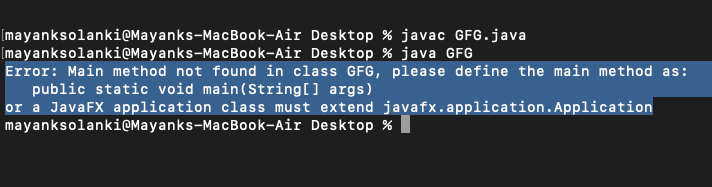Open in App
Not now

# Static Blocks in Java

• Difficulty Level : Easy
• Last Updated : 10 May, 2022

In simpler language whenever we use a static keyword and associate it to a block then that block is referred to as a static block. Unlike C++, Java supports a special block, called a static block (also called static clause) that can be used for static initialization of a class. This code inside the static block is executed only once: the first time the class is loaded into memory.

Calling of static block in java?

Now comes the point of how to call this static block. So in order to call any static block, there is no specified way as static block executes automatically when the class is loaded in memory. Refer to the below illustration for understanding how static block is called.

Illustration:

```class GFG {

// Constructor of this class
GFG() {}

// Method of this class
public static void print() { }

static{}

public static void main(String[] args) {

// Calling of method inside main()
GFG geeks = new GFG();

// Calling of constructor inside main()
new GFG();

// Calling of static block
// Nothing to do here as it is called
// automatically as class is loaded in memory

}
}```

Note: From the above illustration we can perceive that static blocks are automatically called as soon as class is loaded in memory and there is nothing to do as we have to in case of calling methods and constructors inside main().

Can we print something on the console without creating main() method?

It is very important question from the interview’s perceptive point. The answer is yes we can print if we are using JDK version 1.6 or previous and if after that  it will throw an. error.

Example 1-A:  Running on JDK version 1.6 of Previous

## Java

 `// Java Program Running on JDK version 1.6 of Previous`   `// Main class ` `class` `GFG {` `  `  `    ``// Static block` `    ``static` `    ``{` `        ``// Print statement` `        ``System.out.print(` `            ``"Static block can be printed without main method"``);` `    ``}` `}`

Output:

`Static block can be printed without main method`

Example 1-B: Running on JDK version 1.6 and Later

## Java

 `// Java Program Running on JDK version 1.6 and Later`   `// Main class ` `class` `GFG {` `  `  `    ``// Static block` `    ``static` `    ``{` `        ``// Print statement` `        ``System.out.print(` `            ``"Static block can be printed without main method"``);` `    ``}` `}`

Output:Example 1:

## Java

 `// Java Program to Illustrate How Static block is Called`   `// Class 1` `// Helper class` `class` `Test {`   `    ``// Case 1: Static variable` `    ``static` `int` `i;` `    ``// Case 2: non-static variables` `    ``int` `j;`   `    ``// Case 3: Static block` `    ``// Start of static block` `    ``static` `    ``{` `        ``i = ``10``;` `        ``System.out.println(``"static block called "``);` `    ``}` `    ``// End of static block` `}`   `// Class 2` `// Main class` `class` `GFG {`   `    ``// Main driver method` `    ``public` `static` `void` `main(String args[])` `    ``{`   `        ``// Although we don't have an object of Test, static` `        ``// block is called because i is being accessed in` `        ``// following statement.` `        ``System.out.println(Test.i);` `    ``}` `}`

Output

```static block called
10```

Remember: Static blocks can also be executed before constructors.

Example 2:

## Java

 `// Java Program to Illustrate Execution of Static Block` `// Before Constructors`   `// Class 1` `// Helper class` `class` `Test {`   `    ``// Case 1: Static variable` `    ``static` `int` `i;` `    ``// Case 2: Non-static variable` `    ``int` `j;`   `    ``// Case 3: Static blocks` `    ``static` `    ``{` `        ``i = ``10``;` `        ``System.out.println(``"static block called "``);` `    ``}`   `    ``// Constructor calling` `    ``Test() { System.out.println(``"Constructor called"``); }` `}`   `// Class 2` `// Main class` `class` `GFG {`   `    ``// Main driver method` `    ``public` `static` `void` `main(String args[])` `    ``{`   `        ``// Although we have two objects, static block is` `        ``// executed only once.` `        ``Test t1 = ``new` `Test();` `        ``Test t2 = ``new` `Test();` `    ``}` `}`

Output

```static block called
Constructor called
Constructor called```

A class can have any number of static initialization blocks, and they can appear anywhere in the class body. The runtime system guarantees that static initialization blocks are called in the order that they appear in the source code.

Note: We use Initializer Block in Java if we want to execute a fragment of code for every object which is seen widely in enterprising industries in development.